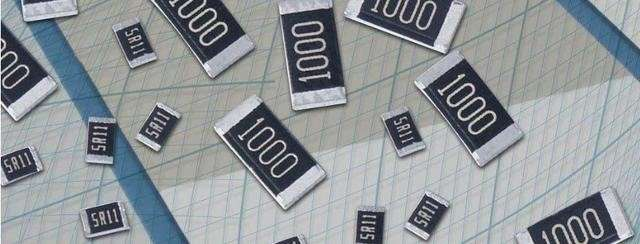# 如何識別晶片電阻阻值與讀法？

## 如何識別晶片電阻阻值與讀法？

Ω= Ω
k = kΩ = 1,000 Ω
M = MΩ = 1,000,000 Ω10×1000=10000Ω=10KΩ
103=10×1000=10000Ω=10KΩ
223=22×1000=22000Ω=22KΩ
122=12×1000=1200Ω=1.2KΩ
301=30×10=300Ω
850=85×（10的零次方=1）=85Ω
1R0=1.0Ω

2.由四個數字組成貼片電阻，表明電阻的誤差±1％。前面三位是有效數字，第四位表示乘零倍數（就是數字是幾，就表示10的幾次方）。例如1502，150是有效數字，直接寫下來，2表示10的二次方。

3.由數字和字母組成貼片電阻阻值讀法，我們只需要把R換成小數點即可。
10R6=10.6R=10.6Ω R12=0.12R=0.12Ω

 代碼為3位數精度5%數字代碼=電阻阻值 代碼為3位數精度5%數字代碼=電阻阻值 代碼為3位數精度5%數字代碼=電阻阻值 代碼為3位數精度5%數字代碼=電阻阻值 1R1=0.1Ω R22=0.22Ω R33=0,33Ω R47=0.47Ω R68=0.68Ω R82=0.82Ω 1R0=1Ω 1R2=1.2Ω 2R2=2.2Ω 3R3=3.3Ω 2R7=4.7Ω 5R6=5.6Ω 6R8=6.8ΩΩ 8R2=8.2Ω 100=10Ω 120=12Ω 150=15Ω 180=18Ω 220=22Ω 270=27Ω 330=33Ω 390=39Ω 470=47Ω 560=56Ω 680=68Ω 820=82Ω 101=100Ω 121=120Ω 151=150Ω 181=180Ω 221=220Ω 271=270Ω 331=330Ω 391=390Ω 471=470Ω 561=560Ω 681=680Ω 821=820Ω 102=1KΩ 122=1.2KΩ 152=1.5KΩ 182=1.8KΩ 222=2.2KΩ 272=2.7KΩ 332=3.3KΩ 392=3.9KΩ 472=4.7KΩ 562=5.6KΩ 682=6.8KΩ 822=8.2KΩ 103=10KΩ 123=12KΩ 153=15KΩ 183=18KΩ 223=22KΩ 273=27KΩ 333=33KΩ 393=39KΩ 473=47KΩ 563=56KΩ 683=68KΩ 823=82KΩ 104=100KΩ 124=120KΩ 154=150KΩ 184=180KΩ 224=220KΩ 274=270KΩ 334=330KΩ 394=390KΩ 474=470KΩ 564=560KΩ 684=680KΩ 824=820KΩ 105=1MΩ 125=1.2MΩ 155=1.5MΩ 185=1.8MΩ 225=2.2MΩ 275=2.7MΩ 335=3.3MΩ 395-3.9MΩ 475=4.7MΩ 565=5.6MΩ 685=6.8MΩ 825=8.2MΩ 106=10MΩ 代碼為4位數精度1%數字代碼=電阻阻值 代碼為4位數精度1%數字代碼=電阻阻值 代碼為4位數精度1%數字代碼=電阻阻值 代碼為4位數精度1%數字代碼=電阻阻值